## 3. 1. 1 Weak Formulation and Galerkin Schemes

The method is based on the notion of the weak formulation or weak solution, which is defined in the following manner: A function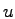is a weak solution of a differential equation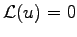within the domain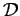, iff for each function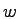the following condition holds true: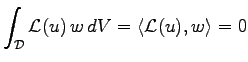(3.1)

This condition can not hold true for arbitrary functions, because the underlying function space does not necessarily provide a weak solution. Consequently, one attempts to fulfill such a condition as well as possible. For this reason, a space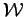of special weighting functions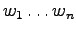is introduced, which is used to measure the deficiency of the numerical solution.

A widely used approach, which uses the shape functions as weighting functions is the Galerkin approach. It has been shown that such an approach has many advantages such as providing a symmetric equation system or system matrix.

The typical formulation of a differential equation using the Galerkin finite element method is written as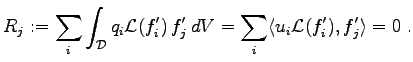(3.2)

The coefficients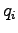denote the weighting coefficients for the shape functions. The solution function can be written in terms of the shape functions in the following manner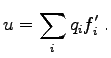(3.3)

It is assumed that the space of possible solution functions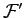is derived from a given tesselation of the simulation domain as shown in Chapter 2. For the sake of simplicity linear shape functions are used, where single shape functions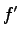collocate with vertices of the tesselation of the simulation domain.

Michael 2008-01-16